Courses

Test: Structure of the Atom- Case Based Type Questions

12 Questions MCQ Test Science Class 9 | Test: Structure of the Atom- Case Based Type Questions

Description
Attempt Test: Structure of the Atom- Case Based Type Questions | 12 questions in 24 minutes | Mock test for Class 9 preparation | Free important questions MCQ to study Science Class 9 for Class 9 Exam | Download free PDF with solutions
QUESTION: 1

Direction: The electronic configuration of an element ‘X’ is 2, 8, 2 :Q. How many Valence electrons are there in element X?

Solution: The element present in the outermost shell are known as Valence electrons. In the outermost shell of element X 2 Valence electrons are present.
QUESTION: 2

Direction: The electronic configuration of an element ‘X’ is 2, 8, 2 :Q. The number of electrons present in the atom of element ‘X’ is __________.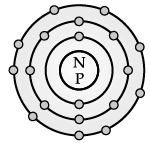Solution: The electronic configuration of element X is 2, 8, 2. So its atomic number is 12 and the number of electrons is equal to the atomic number.
QUESTION: 3

Direction: The electronic configuration of an element ‘X’ is 2, 8, 2 :Q. The Valency of element X is:

Solution: The number of electrons present in the outermost shell is 2. So, the valency will be 2.
QUESTION: 4

Direction: The electronic configuration of an element ‘X’ is 2, 8, 2 :

Q. The element X is:

Solution: Element X is a metal.
QUESTION: 5

Direction: In the following table the mass number and the atomic number of certain elements are given. Study the given data and answer the following questions :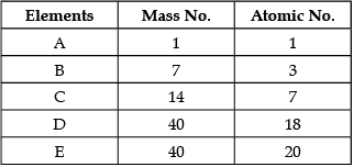Q. The element which can form a cation:

Solution: Element B has number of protons greater than number of electrons.
QUESTION: 6

Direction: In the following table the mass number and the atomic number of certain elements are given. Study the given data and answer the following questions :Q. Any two elements are Isobars:

Solution: The elements having different atomic numbers but same mass numbers are called Isobars. Element D and E both have same mass number i.e. 40 but different atomic number.
QUESTION: 7

Direction: In the following table the mass number and the atomic number of certain elements are given. Study the given data and answer the following questions :Q. Which element will form an anion?

Solution: Element A has number of electrons greater than number of protons.
QUESTION: 8

Direction: In the following table the mass number and the atomic number of certain elements are given. Study the given data and answer the following questions :Q. Which element is a noble gas?

Solution: The atomic number of element D is 18. So, it’s electronic configuration will be 2, 8, 8. The outermost shell is complete, so, it is a noble gas.
QUESTION: 9

In order to overcome the objections raised against Rutherford’s model of the atom, Neil Bohr put forward the following postulates about the model of an atom.

Q. Who amended Rutherford’s short comings?

Solution: Neils’ Bohr amended Rutherford’s shortcomings.
QUESTION: 10

In order to overcome the objections raised against Rutherford’s model of the atom, Neil Bohr put forward the following postulates about the model of an atom.

Q. Atoms are made up of ______, _____ and ______.

Solution: Atoms are made up of fundamental particles and these fundamental particles are electrons, protons and neutrons.
QUESTION: 11

In order to overcome the objections raised against Rutherford’s model of the atom, Neil Bohr put forward the following postulates about the model of an atom.

Q. Atomic mass is the sum of:

Solution: Atomic mass = number of protons + number of neutrons.
QUESTION: 12

In order to overcome the objections raised against Rutherford’s model of the atom, Neil Bohr put forward the following postulates about the model of an atom.

Q. The number of electrons that K-shell and L-shell can accommodate: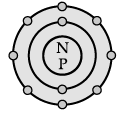Solution: According Bohr’s rule 2n2, first shell or K-shell should accommodate 2 electrons and second shell or L-shell should accommodate 8 electrons.Use Code STAYHOME200 and get INR 200 additional OFF Use Coupon Code

Track your progress, build streaks, highlight & save important lessons and more!

Similar Content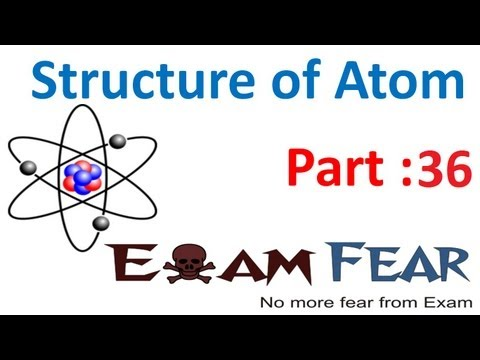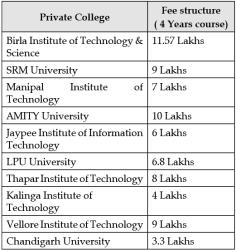Related tests